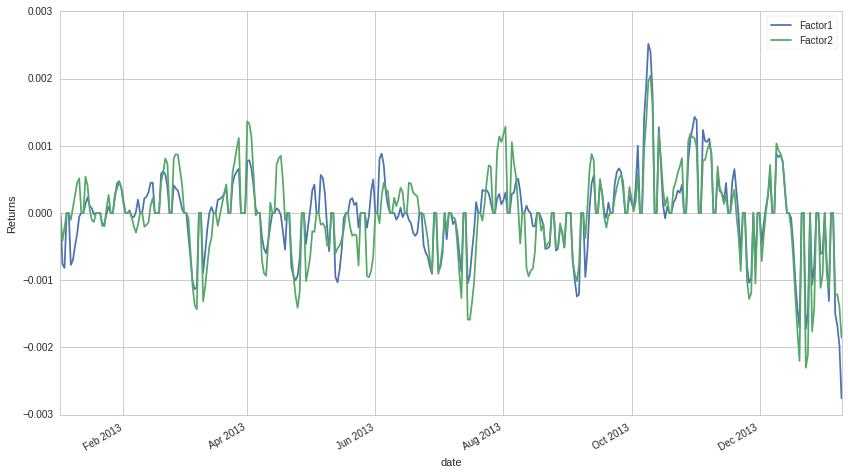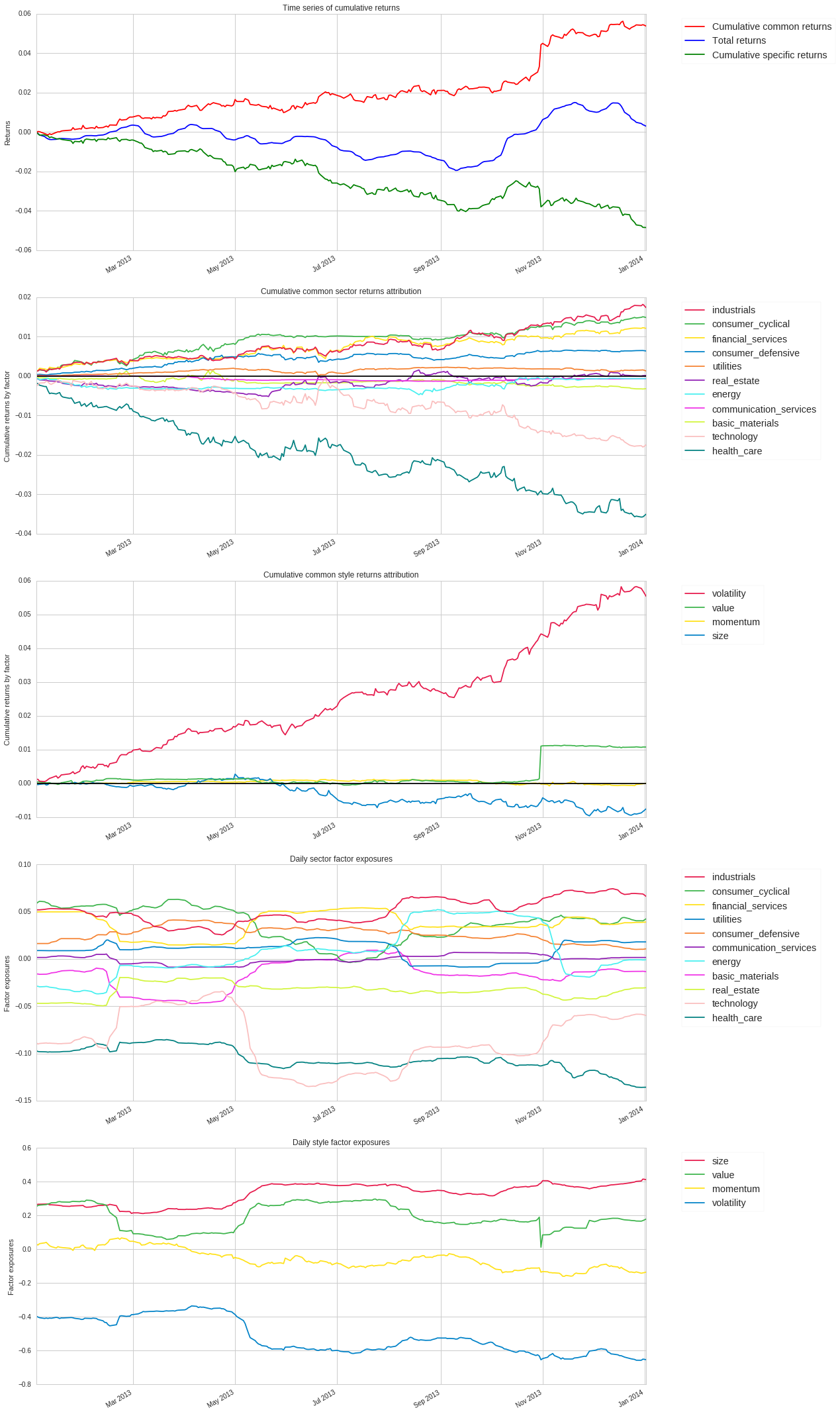Notebook

# Checking Factor Correlation and Risk Exposure¶

by Delaney Mackenzie

Much of the code in this notebook comes from exmamples written by Luca.

This is a quick example notebook showing how to check factor correlation. We start by computing our factors, then we construct portfolios based on the top and bottom quintiles. Then we check the correlation of returns of those portfolios.

This notebook assumes that you have a working knowledge of research and Alphalens. If you don't, check out this tutorial.

These cells can take a little while to run, and the time is dependent on the complexity of your factors and the length of the time window you choose.

In :
from quantopian.pipeline.factors import CustomFactor
from quantopian.pipeline.data.builtin import USEquityPricing

import numpy as np
import pandas as pd
import scipy.stats as stats


Import the Quantopian Tradeable Universe for US stocks.

In :
from quantopian.pipeline.filters import QTradableStocksUS

In :
universe = QTradableStocksUS()


Define our pipeline with the factors you want. We have some fairly simple corporate fundamentals based factors. You might want to use Custom Factors instead.

In :
from quantopian.research import run_pipeline
from quantopian.pipeline import Pipeline
from quantopian.pipeline.classifiers.fundamentals import Sector
from quantopian.pipeline.data import Fundamentals

In :
value = Fundamentals.ebit.latest / Fundamentals.enterprise_value.latest
quality = Fundamentals.roe.latest
pipe = Pipeline(
columns = {
'factor1' : value,
'factor2' : quality,
'Sector' : Sector(mask=universe), # optional, useful to compute individual sector statistics
},
screen=universe
)


Let's run our pipeline to get the daily values of each factor over a set time period.

Since computing factor(s) over a long period of time requires lots of memory, we use the chunksize argument to split our Pipeline computation into a specific number of days, limiting memory usage.

In :
factors = run_pipeline(pipe, '2013-01-01', '2014-01-01', chunksize=250) # chunksize is optional
factors = factors.dropna()


Find all the assets that were ever touched by these factors.

In :
asset_list = factors.index.levels


Get pricing data for these assets.

In :
prices = get_pricing(asset_list, start_date='2013-01-01', end_date='2014-02-01', fields='open_price')


Initialize Alphalens and compute all the forward returns and how they relate to the factor values. This is the first step when running Alphalens to determine how predictive each factor is of forward returns, we'll diverge after this step.

In :
import alphalens as al

sector_labels = dict(Sector.SECTOR_NAMES)
sector_labels[-1] = "Unknown" # no dataset is perfect, better handle the unexpected

In :
factor1_data = al.utils.get_clean_factor_and_forward_returns(
factor=factors["factor1"],
prices=prices,
groupby=factors["Sector"],
quantiles=5,
periods=(1, 5, 10)
)

factor2_data = al.utils.get_clean_factor_and_forward_returns(
factor=factors["factor2"],
prices=prices,
groupby=factors["Sector"],
quantiles=5,
periods=(1, 5, 10)
)

Dropped 0.0% entries from factor data: 0.0% in forward returns computation and 0.0% in binning phase (set max_loss=0 to see potentially suppressed Exceptions).
max_loss is 35.0%, not exceeded: OK!
Dropped 0.0% entries from factor data: 0.0% in forward returns computation and 0.0% in binning phase (set max_loss=0 to see potentially suppressed Exceptions).
max_loss is 35.0%, not exceeded: OK!


Use built-in functionality to compute the returns were you invested long the highest ranked assets and short the bottom ranked assets for each factor. This is standard methodology, but keep in mind that the returns can be pretty heavily affected by the choices you make on how to construct the portfolio from the factor values.

In :
factor1_returns, factor1_positions, factor1_benchmark = \
al.performance.create_pyfolio_input(factor1_data,
period='5D',
capital=1000000,
long_short=True,
group_neutral=False,
equal_weight=True,
quantiles=[1,5],
groups=None,
benchmark_period='1D')

factor2_returns, factor2_positions, factor2_benchmark = \
al.performance.create_pyfolio_input(factor2_data,
period='5D',
capital=1000000,
long_short=True,
group_neutral=False,
equal_weight=True,
quantiles=[1,5],
groups=None,
benchmark_period='1D')


Plot the returns.

In :
import matplotlib.pyplot as plt

In :
factor1_returns.plot()
factor2_returns.plot()
plt.ylabel('Returns')
plt.legend(['Factor1', 'Factor2']);Looks a little correlated, let's check.

In :
np.corrcoef([factor1_returns, factor2_returns])

Out:
array([[ 1.        ,  0.84101734],
[ 0.84101734,  1.        ]])

Correlation is 0.84. This method can be extended to N factors by just adding them appropriately in the code above.

## Risk Exposure¶

Now we'll check the exposure of Factor 1 to the Quantopian risk model factors via Pyfolio. This needs a few pieces of information on risk factor loadings that we'll generate here. To check the exposure of Factor 2 you can just change the code to reference factor2 instead.

In :
import pyfolio as pf

asset_list = factor1_data.index.levels.unique()
start_date = factor1_data.index.levels.min()
end_date   = factor1_data.index.levels.max()

factor_returns = get_factor_returns(start_date, end_date)


In :
pf.tears.create_perf_attrib_tear_sheet(factor1_returns,
positions=factor1_positions,
factor_returns=factor_returns,
pos_in_dollars=True)

/usr/local/lib/python2.7/dist-packages/pyfolio/perf_attrib.py:589: UserWarning: Could not find factor loadings for 120 dates: (first missing is 2013-01-05 00:00:00+00:00, last missing is 2014-01-09 00:00:00+00:00). Truncating date range for performance attribution.
warnings.warn(warning_msg)


## Performance Relative to Common Risk Factors¶

Summary Statistics
Annualized Specific Return -4.84%
Annualized Common Return 5.35%
Annualized Total Return 0.29%
Specific Sharpe Ratio -2.58
Exposures Summary Average Risk Factor Exposure Annualized Return Cumulative Return
basic_materials -0.02 -0.32% -0.32%
consumer_cyclical 0.04 1.48% 1.48%
financial_services 0.04 1.20% 1.21%
real_estate -0.03 0.01% 0.01%
consumer_defensive 0.03 0.63% 0.64%
health_care -0.11 -3.49% -3.50%
utilities 0.01 0.13% 0.13%
communication_services -0.00 -0.07% -0.07%
energy 0.01 -0.07% -0.07%
industrials 0.05 1.73% 1.73%
technology -0.09 -1.74% -1.75%
momentum -0.06 0.01% 0.01%
size 0.33 -0.75% -0.75%
value 0.19 1.07% 1.08%
short_term_reversal -0.05 0.12% 0.12%
volatility -0.52 5.51% 5.54%This presentation is for informational purposes only and does not constitute an offer to sell, a solicitation to buy, or a recommendation for any security; nor does it constitute an offer to provide investment advisory or other services by Quantopian, Inc. ("Quantopian"). Nothing contained herein constitutes investment advice or offers any opinion with respect to the suitability of any security, and any views expressed herein should not be taken as advice to buy, sell, or hold any security or as an endorsement of any security or company. In preparing the information contained herein, Quantopian, Inc. has not taken into account the investment needs, objectives, and financial circumstances of any particular investor. Any views expressed and data illustrated herein were prepared based upon information, believed to be reliable, available to Quantopian, Inc. at the time of publication. Quantopian makes no guarantees as to their accuracy or completeness. All information is subject to change and may quickly become unreliable for various reasons, including changes in market conditions or economic circumstances.